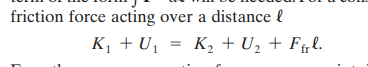# Conservation of energy problem with friction included

• simphys

#### simphys

Homework Statement
A 96-kg crate, starting from rest, is pulled across a floor
with a constant horizontal force of 350 N. For the first 15 m
the floor is frictionless, and for the next 15 m the coefficient
of friction is 0.25. What is the final speed of the crate?
Relevant Equations
conservation of energy
so I haven't looked at the solution yet, but I know that a 100% the velocity needs to be bigger, but analytically, I get a - sign instead of a + sign as you'll see at the final square root.

So for the first 15meters of the motion all you should know is that ##v_1 = 10.458 m/s##.

for the 2nd part:
energy is conserved right. 2 NC-forces are acting on it but net force is positive however.
So ##E_1 = E_2 + W_{NC}## (1)
##W_{NC} = F{net,NC}*\Delta x##

to get ##F_{net,NC}## , we have ##F = 350N## and the friction force is found from an FBD
so ##F_r = \mu_kN = \mu_kmg##
##F_{net,NC} = F - \mu_kmg = 350 - 0.25*96*9.81 = 114.56N##
Now back to ##(1)##:
##\frac12mv_1^2 = \frac12mv_2^2 + F_{net,NC}\Delta x## where work done by the NC forces is pos.
rearranging to ##v_2##, we get:
##v_2 = \sqrt{\frac12mv_1^2 - F_{net,NC}\Delta x}## WHERE i GET A - SIGN INSTEAD OF A + SIGN HERE, WHY?
##v_2 = 8.6m/s##

Homework Statement:: A 96-kg crate, starting from rest, is pulled across a floor
with a constant horizontal force of 350 N. For the first 15 m
the floor is frictionless, and for the next 15 m the coefficient
of friction is 0.25. What is the final speed of the crate?
Relevant Equations:: conservation of energy

WHY?
Because you put the term on the wrong side of the equation. The work-energy theorem states that the difference between kinetic energy after and before equals the work done.

However, you are needlessly complicating things here by computing the intermediate velocity. You have two forces, the pulling force and the friction force. The first is constant and acts over a total of 30 m. The second is also constant (once applied) and acts over a total of 15 m. You can easily compute the total work done from when the crate was at rest.

•simphys
Because you put the term on the wrong side of the equation. The work-energy theorem states that the difference between kinetic energy after and before equals the work done.

However, you are needlessly complicating things here by conputing the intermediate velocity. You have two forces, the pulling force and the friction force. The first is constant and acts over a total of 30 m. The second is also constant (once applied) and acts over a total of 15 m. You can easily compute the total work done from when the crate was at rest.
Oh gosh, I just thought of it myself that it could've prob been done by considering like that, but then I thought nehh we have two different forces, but of course you are totally right, thank you!

And okay... thank you I wasn't really sure which equatoin the actual statement for conserv of E would be. That's why I stated that one aka ##E_1 = E_2 + W_{NC}##, so I'll just use ##\delta K = W_C + W_{NC}## from now on, thank you.

See @Orodruin it's soo confusing in this book...I mean c'mon now...

they'd be better off stating more generally ##\Delta K = W_{net}##